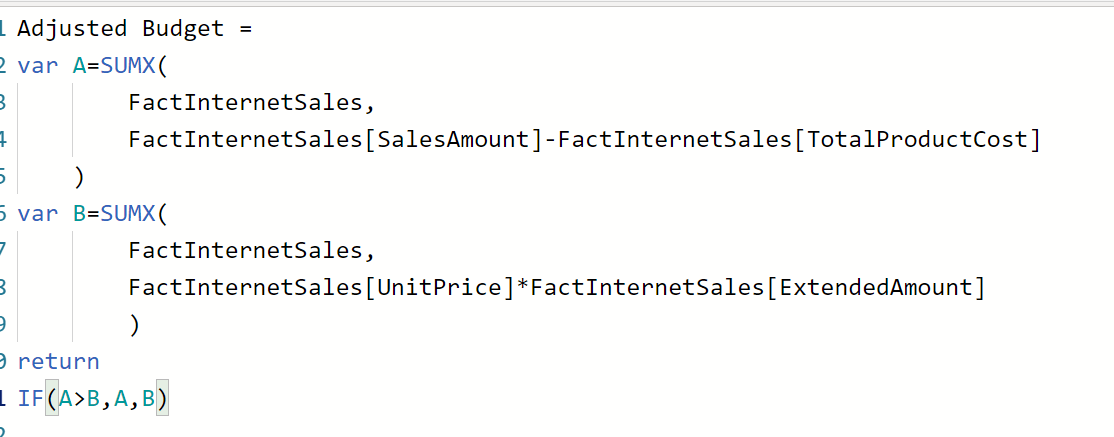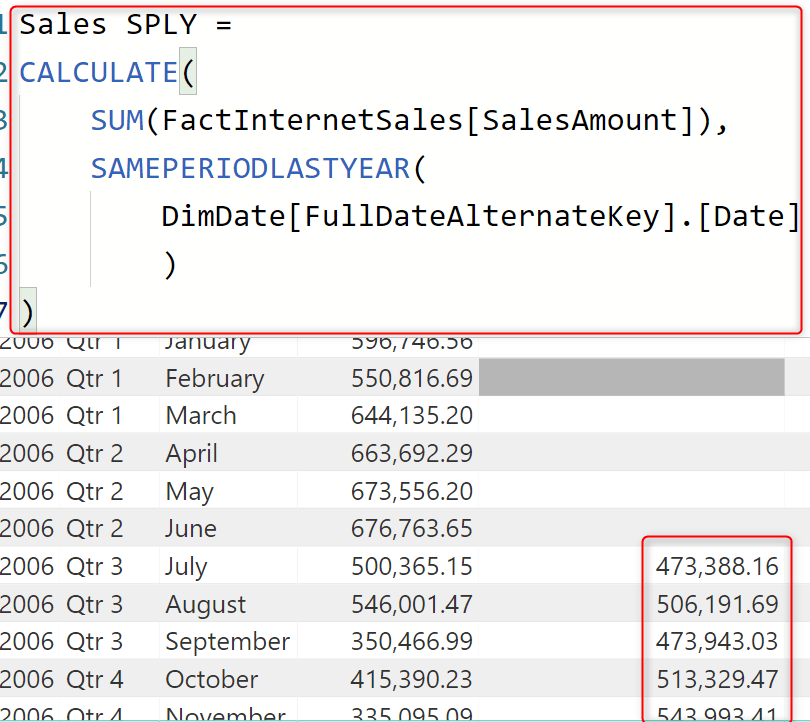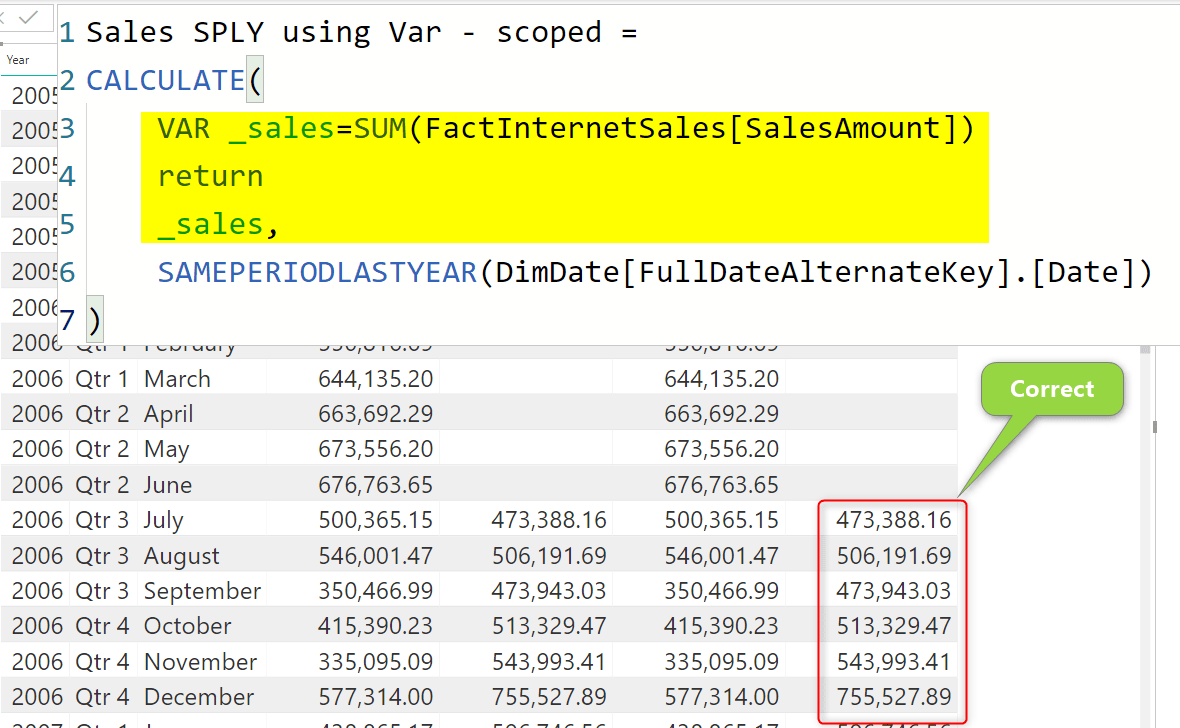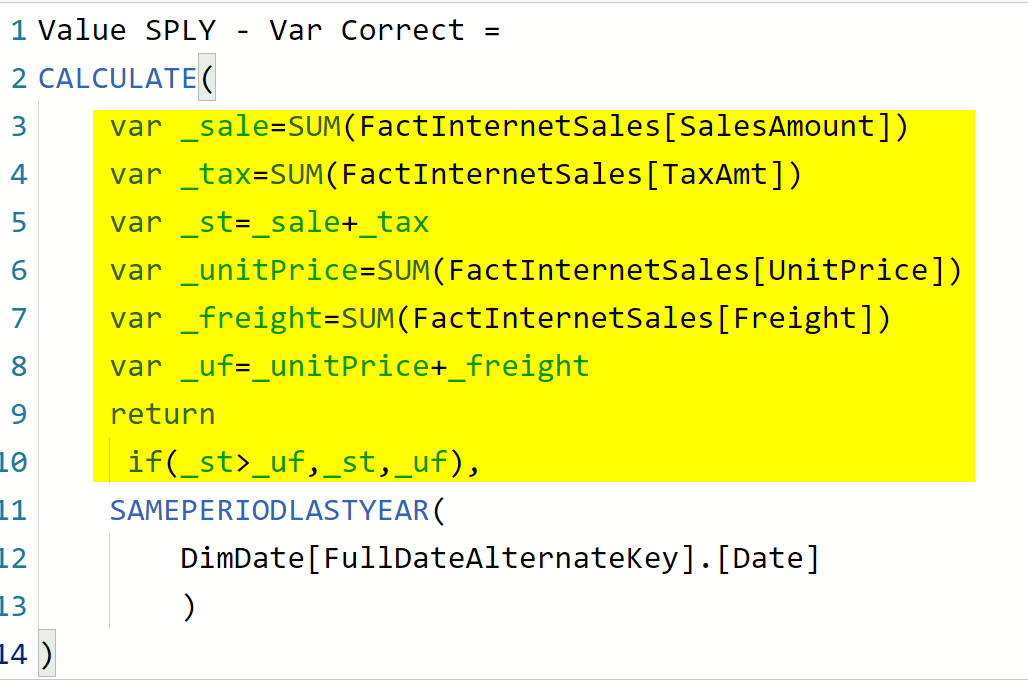# Caution When Using Variables in DAX and Power BII have written another blog post about variables previously and explained how useful variables are. Variables in DAX are helpful in both readability and also the performance of your code. However, there are scenarios that you have to be careful when you use variables. Because variables are stored, they might return a result that is different from if you had that definition in a measure. Let’s see an example in this blog post.

## Variables in DAX

You can define a DAX variable using VAR statement, and then use it in a RETURN statement, or even in another variable through that expression. Here, for example, you can see a use case of the variable:The above is an example of the “right” usage of the variable. Variable can be used mistakenly in a wrong situation though. Let’s see an example. If you want to learn what are variables and how they can make your DAX expressions better, read my blog article here.

## Calculating a Value Before Filtering

One of the most common mistakes for using variables is in a scenario that you calculate the value before the custom filter applied. Let’s assume you want to calculate the same period last year sales amount, the calculation normally is like below: (If you like to learn how to calculate the same period last year value, read my blog post here)

```Sales SPLY =
CALCULATE(
SUM(FactInternetSales[SalesAmount]),
SAMEPERIODLASTYEAR(DimDate[FullDateAlternateKey].[Date])
)```For the expression above, you don’t need a variable, but let’s say even for that expression if you decided to create a variable for the expression part of it, it looks like below;

```Sales SPLY using Var =
VAR _sales=SUM(FactInternetSales[SalesAmount])
return
CALCULATE(
_sales,
SAMEPERIODLASTYEAR(DimDate[FullDateAlternateKey].[Date])
)```

As you can see in the below screenshot, the result is wrong! The result is similar to the sales amount of each month rather than the sale of the same period last year!## Why?

In DAX, variables are calculated within the scope in which they are written, and then the result of them is stored and used in the rest of the expression.

This means that when we create the variable as VAR _sales=SUM(FactInternetSales[SalesAmount]), the expression runs on the filter context of the visual, which is the month in which the calculation is evaluated. As a result. for each month, the value of _sales would be the sum of the SaleAmount column in that month, then the rest of the expression is using the stored result from that point onward.

Because the value of the variable is stored, it is better that you calculate it considering all the filters that you want to apply.

In this case, the context of the filter which we are trying to add is the SAMEPERIODLASTYEAR(DimDate[FullDateAlternateKey].[Date]), and we want the variable to be calculated using this filter context.

So calculating the variable in this scenario before the CALCULATE statement, make it wrong. You either have to not use a variable here for this scenario, or use it in the context of that filter, which is possible with the change in SCOPE of the variable;

```Sales SPLY using Var - scoped =
CALCULATE(
VAR _sales=SUM(FactInternetSales[SalesAmount])
return
_sales,
SAMEPERIODLASTYEAR(DimDate[FullDateAlternateKey].[Date])
)```

This expression is using the variable inside the context that it should be calculated, and as a result, it returns the correct output;## Another Example

The example you have seen is more like a “learning” example because you won’t create a variable usually for such a simple statement. I used that example to explain to you what is the problem, and how to solve it. Now, here is another example, which makes sense more;Then this would be the wrong usage of variables for it:

## Wrong Use of Variables

The expression below returns a different result from the above expression because all the variable values are calculated outside of the CALCULATE expression and the filter of SAMEPERIODLASTYEAR.## The Right Way

The expression below, however, is correct, because the variables are used in the scope of the context of the filter needed to be applied:Here is the result and comparison of the two different expressions:## Summary

You might say that there are other ways of writing this expression without the need for variables, and you are right. The purpose of this post, however, was to teach you, that although variables can be used anywhere, however, their result might not be always the same. Because they are evaluated in the context in which they are written. The scope that you write the variable is important and should be used cautiously.

## Video

Trainer, Consultant, Mentor
Reza Rad is a Microsoft Regional Director, an Author, Trainer, Speaker and Consultant. He has a BSc in Computer engineering; he has more than 20 years’ experience in data analysis, BI, databases, programming, and development mostly on Microsoft technologies. He is a Microsoft Data Platform MVP for 12 continuous years (from 2011 till now) for his dedication in Microsoft BI. Reza is an active blogger and co-founder of RADACAD. Reza is also co-founder and co-organizer of Difinity conference in New Zealand, Power BI Summit, and Data Insight Summit.
Reza is author of more than 14 books on Microsoft Business Intelligence, most of these books are published under Power BI category. Among these are books such as Power BI DAX Simplified, Pro Power BI Architecture, Power BI from Rookie to Rock Star, Power Query books series, Row-Level Security in Power BI and etc.
He is an International Speaker in Microsoft Ignite, Microsoft Business Applications Summit, Data Insight Summit, PASS Summit, SQL Saturday and SQL user groups. And He is a Microsoft Certified Trainer.
Reza’s passion is to help you find the best data solution, he is Data enthusiast.
His articles on different aspects of technologies, especially on MS BI, can be found on his blog: https://radacad.com/blog.

## 2 thoughts on “Caution When Using Variables in DAX and Power BI”

•MF says:

Nice post and clear explanation!
I ran into similar misleading before and it took me quite a while to debug. But that’s a good learning exercise! 😅

•Hoss Satour says:

Great article. Thank you very much Reza Rad